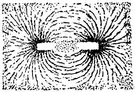# magnetomotive force

Also found in: Thesaurus, Acronyms, Encyclopedia, Wikipedia.

## mag·ne·to·mo·tive force

(măg-nē′tō-mō′tĭv)
n. Abbr. mmf
The work that would be required to carry a hypothetical isolated magnetic pole of unit strength completely around a magnetic circuit.

## magnetomotive force

n
(General Physics) the agency producing a magnetic flux, considered analogous to the electromotive force in an electric circuit; equal to the circular integral of the magnetic field strength. Symbol: F

## mag•ne′to•mo′tive force′

(mægˈni təˈmoʊ tɪv, -ˌni-)

n.
a scalar quantity that is a measure of the sources of magnetic flux in a magnetic circuit.

ThesaurusAntonymsRelated WordsSynonymsLegend:
 Noun 1magnetomotive force - the force that produces magnetic fluxforce - (physics) the influence that produces a change in a physical quantity; "force equals mass times acceleration"
References in periodicals archive ?
(vii) The magnetomotive force created by each phase of the two frames is sinusoidal distribution.
Variation of magnetomotive force in the half-core window area
MMF: Magnetomotive force provided by PM, A * turn [R.sub.l]: Magnetic reluctance of the rod, H [R.sub.g]: Magnetic reluctance of the air gap from GMM bar to wall, H [R.sub.w]: Magnetic reluctance of wall, H [phi]: Magnetic fluxes, wb A: Magnetic vector potential, wb/m [phi]: Magnetic scalar potential, wb/m [H.sub.i]: Magnetic field in each element, A/m [H.sub.avg]: Average magnetic field, A/m [L.sub.GMM]: Total length of GMM patches, m [L.sub.PM]: Total length of PM patches, m [lambda]: Magnetostriction of GMM [[lambda].sub.s]: Saturated magnetostriction of GMM [M.sub.s]: Saturated magnetization of GMM, A/m M: Magnetization of GMM, A/m [A.sub.GMM]: Section area of GMM rod, [m.sup.2].
Magnetomotive force (MMF) distribution in the air gap produced by a current [i.sub.a] flowing in any coil a (stator or rotor windings) is as follows:
For this case, 260 Amp-turns constitute the coil magnetomotive force. The simulation results are shown in Figure 3, which is a cross-section of the B-field strength in the plane of the gap.
Where, [N.sub.f] and [N.sub.s] are effective turns of the field windings and the stator windings, respectively; [F.sub.f] is the magnetomotive force; R is the reluctance of the flux line direction and M is the mutual induction between rotor and stator windings .
Despite the differences of poles number, slots number, and the coiling form of the 3-phase AC motor, the physical model of stator can be described as in Figure 1 for the symmetry of the magnetic circuit and the magnetomotive force (MMF) generated by powered winding.
The fundamental harmonic of magnetomotive force (mmf) created by each rotor bar is:
and the calculated magnetomotive force of permanent magnets by rated temperature
The value of the magnetomotive force (MMF) [F.sub.[delta]] of the stator winding, which causes the value of magnetic induction in the air gap depends on the value of the stator current I flowing through the N turns of the coils of the stator winding.
The leakage inductance is proportional to magnetomotive force between the windings that acts in air gap of core  (Fig.
Specifically, due to the slot harmonic effect on the magnetic pull  and the elliptical rotating magnetomotive force caused by the asymmetric 3-phase currents , the stator will be dragged into an ellipse form after a long period performance [27,28].

Site: Follow: Share:
Open / Close